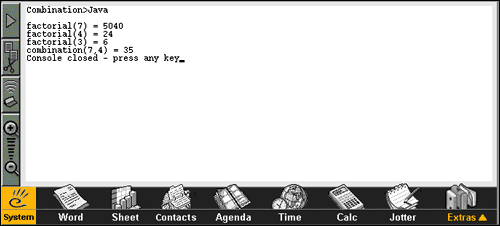Home > Epoc > Samples > Combination > JavaCombination in Java

####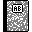Purpose

This is the Java version of our combination application.

####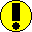Requirements

You need Epoc Java SDK (see tools section).

####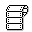Source code

```/*
* Combination.java
* Java
*/

package samples;

/**
* A sample that computes factorial(n) and combination(n,p)
*/
public class Combination
{
private int myN, myP;

public Combination(int n, int p)
{
myN = n;
myP = p;
}

public long factorial(int n)
{
return (n <= 1) ? 1 : n*factorial(n-1); // he-he... let's mix a ternary operator (?) and recursion...
}

public long combination(int n, int p)
{
return factorial(n) / (factorial(n - p) * factorial(p));
}

public void go()
{
System.out.println("Combination>Java");
System.out.println();
System.out.println("factorial(" + myN + ") = " + factorial(myN));
System.out.println("factorial(" + myP + ") = " + factorial(myP));
System.out.println("factorial(" + (myN-myP) + ") = " + factorial(myN-myP));
System.out.println("combination(" + myN + "," + myP + ") = " + combination(myN, myP));
}

public static void main(String[] args)
{
if (args.length != 2)
{
System.err.println("Usage: Combination n p");
System.exit(0);
}

int argN = java.lang.Integer.parseInt(args),
argP = java.lang.Integer.parseInt(args);

Combination comb = new Combination(argN, argP);
comb.go();
}
}
```

Here's the .txt file that contains arguments to fire the JVM (classpath, class name and class args):

`-cp combination.jar samples.Combination 7 4`

Here's the .lst file used by bmconv to generate the .mbm (Multi-bitmap) from the collection of .bmp: (Combination16i.bmp is the 16x16 icon, Combination16m.bmp is the transparency mask to apply to the same icon for drawing, Combination24i.bmp is the 24x24 icon, etc):

```Combination.mbm

/c4Combination16i.bmp
Combination16m.bmp
/c4Combination24i.bmp
Combination24m.bmp
/c4Combination32i.bmp
Combination32m.bmp
/c4Combination48i.bmp
Combination48m.bmp
```

And here's the .rss to generate the .aif with aiftool! (Phew...)

```// Combination.rss
// Epoc
// Java
#include <aiftool.rh>

RESOURCE AIF_DATA
{
app_uid=0x01234567;
//
caption_list=
{
CAPTION { code=ELangEnglish; caption="Combination"; },
CAPTION { code=ELangFrench; caption="Combinaison"; },
CAPTION { code=ELangGerman; caption="Kombination"; },
CAPTION { code=ELangSpanish; caption="Combinación"; }
};
//
num_icons=4;
//
embeddability=KAppNotEmbeddable;
}
```

####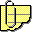Comments

See the magic of Java:

1. We are using exactly the same Java source code as for other platforms
2. Same remark for the byte-code! Not even a single recompilation!!!
3. You may install the byte code (along with its accompanying files) either on WINS emulator or on a real device. No need to specify target machine when you compile Java code :)

The core functions are located at the top of the .java file: factorial(), combination() and go(). Be careful, there's also Combination() with capital 'C', but its the constructor!

Here, note that the amount of overhead is about the same as for the C++ version for Epoc.

Install the .jar (jarred-up class), .aif (Application Information File), .app (Links app to its UID), .txt (used to fire the JVM with the right arguments and the right class).

• The make-aif.bat generates the .mbm and .aif
• The collection of .bmp to generate the .mbm